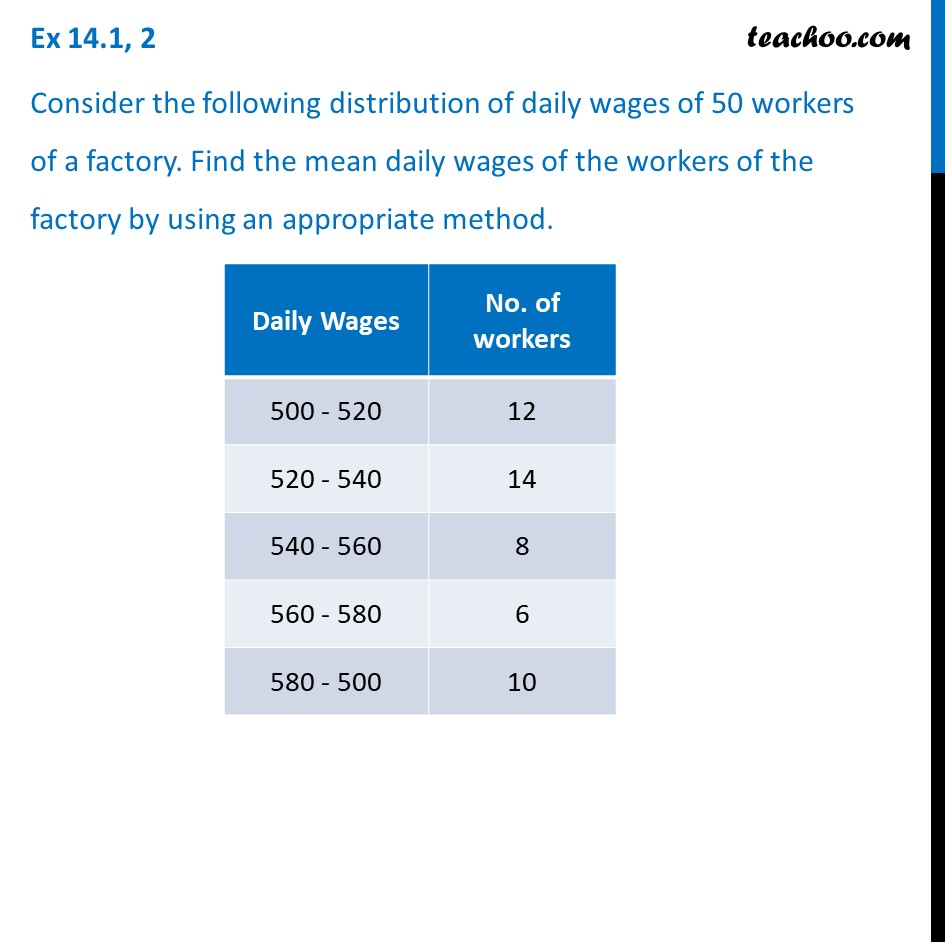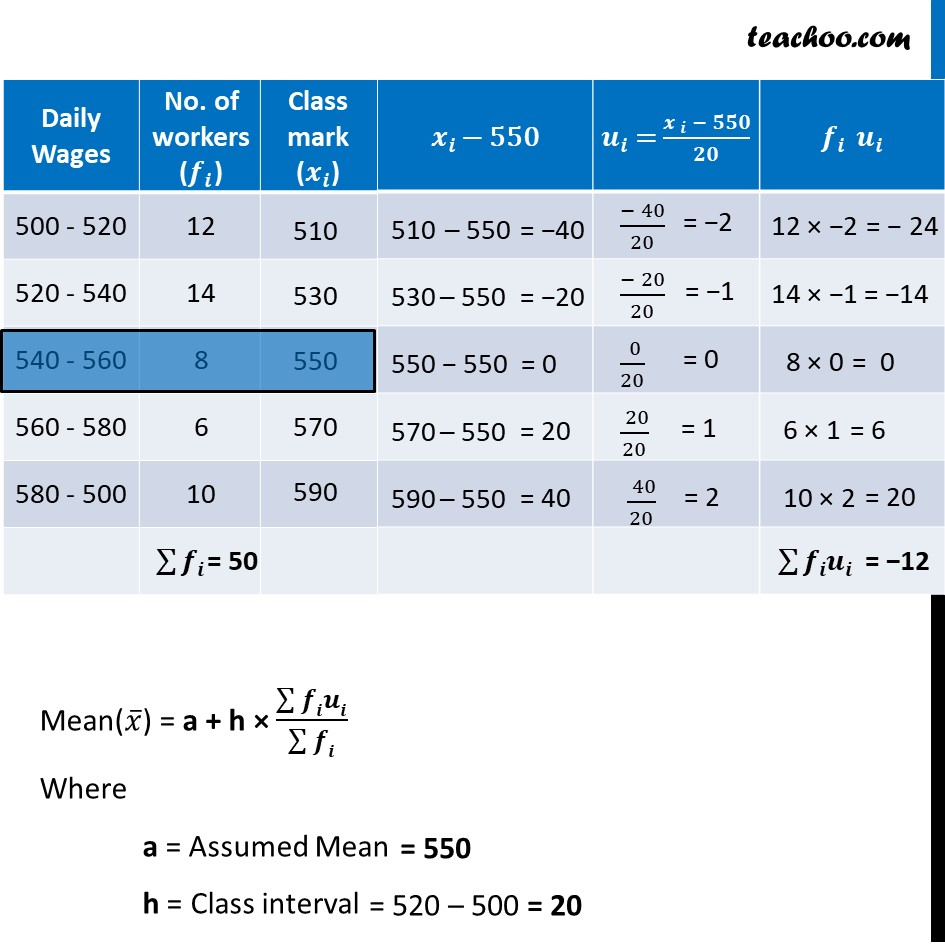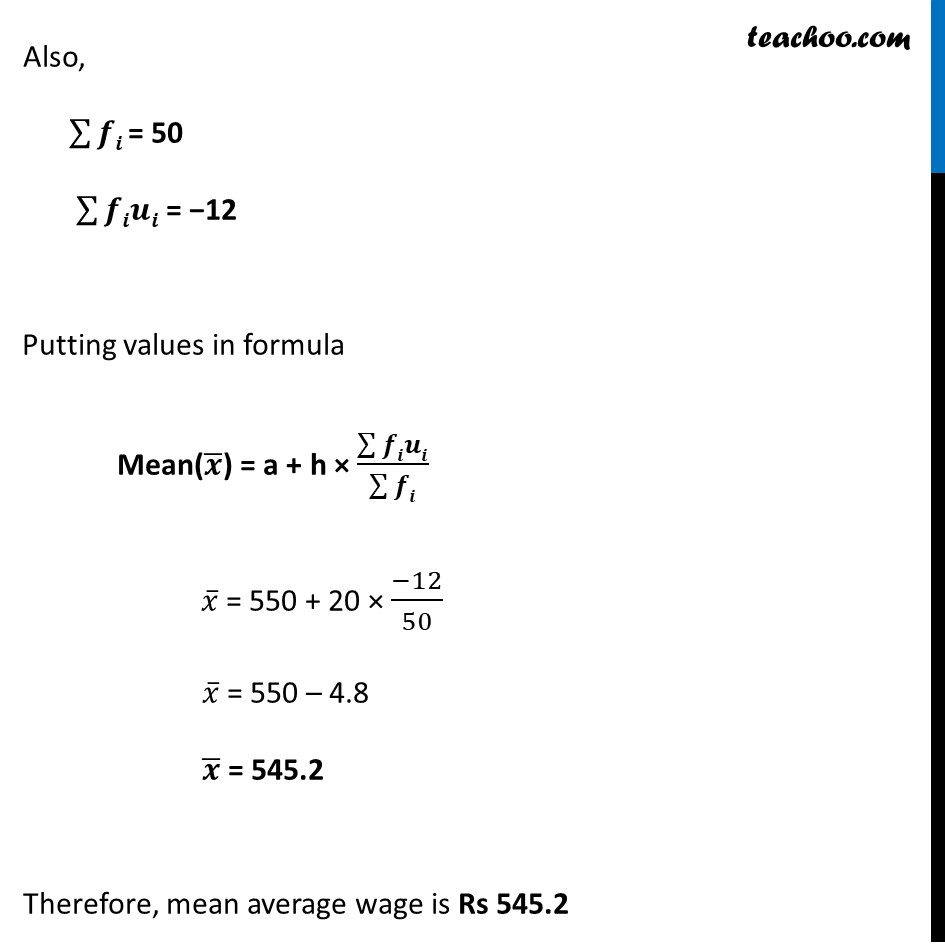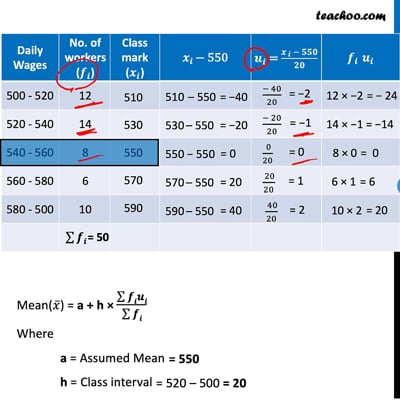Mean

Chapter 14 Class 10 Statistics
Concept wiseThis video is only available for Teachoo black users

Introducing your new favourite teacher - Teachoo Black, at only ₹83 per month

### Transcript

Ex 14.1, 2 Consider the following distribution of daily wages of 50 workers of a factory. Find the mean daily wages of the workers of the factory by using an appropriate method. Mean(𝑥 ̅) = a + h × (∑▒𝒇𝒊𝒖𝒊)/(∑▒𝒇𝒊) Where a = Assumed Mean h = Class interval = 550 = 520 – 500 = 20 Also, ∑▒𝒇𝒊 = 50 ∑▒𝒇𝒊𝒖𝒊 = −12 Putting values in formula Mean(𝒙 ̅) = a + h × (∑▒𝒇𝒊𝒖𝒊)/(∑▒𝒇𝒊) 𝑥 ̅ = 550 + 20 × (−12)/50 𝑥 ̅ = 550 – 4.8 𝒙 ̅ = 545.2 Therefore, mean average wage is Rs 545.2